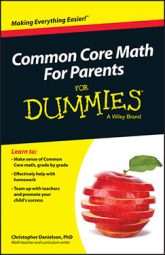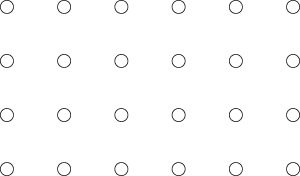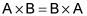##### Common Core Math For Parents For Dummies with Videos OnlineIn Common Core math, third and fourth grade students begin to study multiplication. The foundation for multiplication is equal‐sized groups, whether they are units, pairs, or arrays.

To prepare for this work, second graders identify and work with equal‐sized groups — even before they name the multiplication and division ideas that follow.

When you count groups of things (such as pairs of shoes), you change units. A unit is a thing that you count. You can count the number of shoes (one unit) in your closet, or you can count the number of pairs (a different unit) of shoes. Paying careful attention to units is important in setting the stage for multiplication and for understanding place value.

One of the most important equal groupings is a pair. Children have lots of experience with things that come in twos. Shoes, eyes, and partners in class are familiar examples of pairs to students. Second grade builds on this familiarity by having students:

• Count by twos

• Separate groups of objects into pairs

• Separate groups of objects into two equal‐sized groups

• Decide whether numbers are odd or even

You can help your second grader notice that some things usually come in groups. Give your child practice counting both groups and individual things. Eggs, bicycle wheels, and grapes are all things that usually come in groups. Ask whether these groups are always (or almost always) the same size. For example, eggs almost always come in 12, bicycle wheels almost always come in pairs, but the number of grapes in a bunch can vary widely.

Whether students are studying groups of two or groups of a different size, one of the more useful ways of showing groups is called an array. In math class, an array is a series of things arranged in rows and columns. The figure shows an example of an array.An array of dots.

Arrays are useful because they show two ways of grouping the dots. The array in the figure has four groups of six if you consider the rows, or six groups of four if you consider the columns. In third grade, arrays help justify the commutative property of multiplication — namely, that the order of the numbers you multiply doesn't matter when finding the total.

This distinction is important because when students begin to study multiplication, they think about situations, such as 4 plates of 6 cookies each. It isn't obvious that 4 plates of 6 cookies is the same total number of cookies as 6 plates with 4 cookies each, and the two situations are quite different otherwise. Knowing that A groups of B is always the same amount as B groups of A, sois an important achievement. This insight becomes especially useful as the study of multiplication is extended to multi‐digit numbers in third and fourth grades, to fractions in fifth grade, and to algebra in sixth grade and beyond.